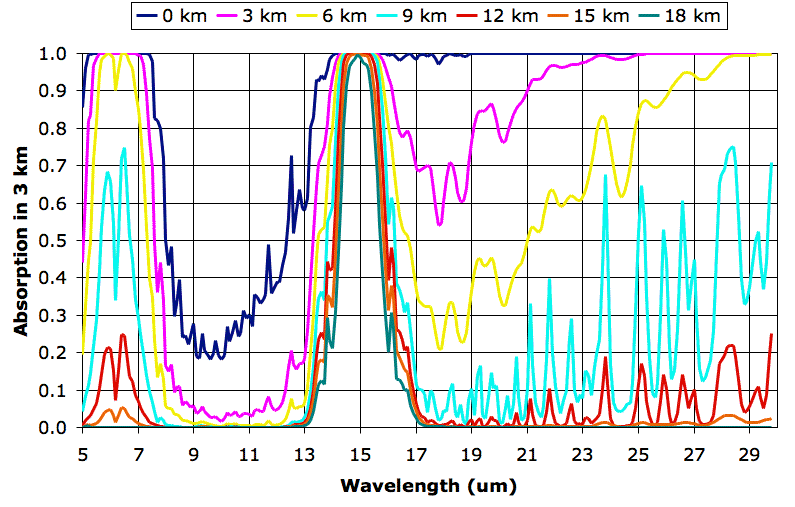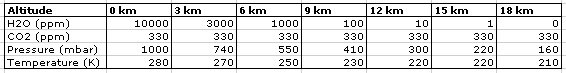## Saturday, July 3, 2010

### Earth's Atmosphere

The following graph shows how various layers of the Earth's atmosphere absorb long-wave radiation. We plot wavelength on the horizontal axis. On the vertical axis we plot the fraction of radiation absorbed along a 3-km path. There are seven absorption plots. Each plot corresponds to a different altitude. The 0-km plot gives the absorption along a 3-km path through a mixture of air and water vapor that is typical at sea-level in the Earth's atmosphere at latitude 30° north. The 3-km plot gives absorption along a 3-km path through a mixture that is typical at altitude 3 km. The final plot gives the absorption along a 3-km path through a mixture typical at 18 km. Click on the graph for a larger version.We obtained the individual plots of absorption along a 3 km path using the Spectral Calculator. We obtained our typical values for temperature and water vapor content from these plots. We obtained the pressure using the approximate formula for atmospheric pressure with altitude that we derived in Atmospheric Pressure. The following table shows the values we used.Each plot serves as an estimate of how much radiation will pass through a 3-km layer of the atmosphere from below. The 0-km plot shows that almost all the long-wave radiation emitted by the Earth is absorbed in the first 3 km of the atmosphere, except for wavelengths between 8 μm and 13 μm. Wavelengths between 8 μm and 13 μm pass all the way through the atmosphere. At altitude 9 km, we see the atmosphere starts to become transparent to wavelengths greater than 16 μm. Absorption between 14 μm and 16 μm at altitude 18 km is due to CO2.

In future posts, we will show how these plots allow us to estimate the altitude of the Earth's tropopause and estimate the effect of CO2 concentration upon the Earth's surface temperature.

1.This is a nice work! I think I'll look over it from the start.

Just a quick question about the radiative transfer: As radiation goes through atmosphere, it is partially thermalized. Basically it means that IR radiation might be converted into thermal movement of molecules. Does the radiative transfer code take this into account? What I mean is that a photon is not simply absorbed then another photon of the same frequency emitted, but for example from one photon you might end up with two at half the energy of the initial one (but of course, things are not that simple). The fact that the radiation is only partially thermalized is not helping, either.

1.Thank you for the compliment.

The term "partially thermalized" does not have any meaning to me, I'm afraid. Under no circumstance is any photon split into two photons. Photons can interact with electrons, imparting some energy and momentum to the electron, and generating a new photon that goes off in a random direction. That's scattering. Scattering happens with blue light, but is negligible at infra-red wavelengths.

Infra-red photons are either absorbed or not absorbed. When an infra-red photon is absorbed, its energy turns into heat.

That said, I believe the answer to your question is yes, our code takes this radiation transfer into account. Eventually, when we get to the simulation of the atmosphere with the Sun warming the Earth and clouds forming and creating rain and snow (see later posts), the atmospheric layers are radiating up and down, and some radiation is absorbed above and below.

2."Infra-red photons are either absorbed or not absorbed. When an infra-red photon is absorbed, its energy turns into heat." - This is what I was talking about. The idea is that the energy that turns into thermal energy won't come back as a photon of the same energy as the incident one, necessarily. It might turn out to come out as two photons of half the original energy (this is simplified). About thermalisation, it is the same meaning as on Wikipedia: http://en.wikipedia.org/wiki/Thermalization Since one does not achieve thermal equilibrium (and indeed that could be only a local idealization - that is cvasi equilibrium for processes on Earth) in the physics, the 'partial thermalisation' I think is correct. Anyway, is of no use to complicate the models too much, you won't get better precision for long.

2.We might assume that the entire atmosphere is in thermal equilibrium. But there is no need to assume that packets or layers of air in the atmosphere are in thermal equilibrium. Indeed: they are not, which is why they heat up near the surface and cool down in the tropopause. So I'm not sure why you are bringing up the fascinating subject of Thermalization.

If a gas can absorb an infra-red photon, this is because it has a vibrational mode that can absorb that particular photon's exact energy. This vibrational mode will subsequently distribute the photon's energy to the surrounding gas. Any one of the gas molecules can, at any time, emit a photon of the same energy from the same vibrational mode. Thus absorption and emission are related through this vibrational mode, but in no way is a molecule that has just absorbed a photon more likely to emit a photon than any other.

Furthermore, if a gas has only one infra-red vibrational mode, then it absorbs and emits only photons of that mode's energy. CO2 has many infra-red vibrational modes, but they are clustered around the 15-um photon wavelength, so CO2 absorbs and emits only around 15 um, and will not emit a photon of half the energy (double the wavelength) as the one it absorbs, no matter how hot it gets. (See Radiative Symmetry.)

Meanwhile, O2 and N2 don't have vibrational modes at infra-red energies, so neither absorb nor emit infrared photons.